## Mathematics Paper 2 Questions - Maranda Mock Examinations 2022

Instructions to Candidates

1. This paper consists of two sections; Section I and Section II.
2. Answer all the questions in Section I and any five questions from Section II
3. Show all the steps in your calculations
4. Marks may be given for correct working even if the answer is wrong.
5. Non-programmable silent electronic calculators and KNEC Mathematical tables may be used, except where stated otherwise.

### QUESTIONS

SECTION I (50 marks)
Answer all questions in this section in the spaces provided

1. A right solid cone has a radius of 10 cm and a slant height of 15 cm. Given that the radius and slant height has error of 0.2% and 0.4% respectively, find the percentage error in finding its surface area correct to 3d.p. (3 marks)
2. Solve for p in (log2p)2 = log2p4 - log28  (3 marks)
3. Evaluate 5cos2ø + 2 = 3sin2ø - 2cosø given that -180º ≤ ø ≤ 180º  (4 marks)
4. In the triangle XYZ, XY = 2 cm, YZ = (2√3 - 1) cm and angle YXZ = 60º. Determine sin (X Z Y), giving your answer in the form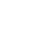, where a and b are integers. (3 marks)
5. The diagram below shows a circle centre O.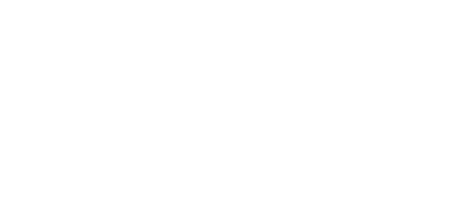A point P lies on the line AO such that a tangent from point P to the circle makes an angle of 300 with the line AO. By construction, locate the point P. (3 marks)
6. Make the subject of the formula in p = (ar2 - s)1/n  (3 marks)
7. Expand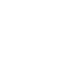in descending powers of , up to the term independent of . If the term independent of is 735, find the value of . (3 marks)
8. A baker bakes two types of cookies, a marmalade cake and sweat loaves of bread. Each day she bakes x marmalade cakes and y sweat loaves of bread. The conditions of the cookies are subject to the following conditions. x > 20, y > 10, 4x + 3y ≥ 120 and 7x + 3y ≤ 280
He makes a profit of Ksh 50 on each marmalade cake and Ksh 60 on each sweat loaf of bread.
1. Graph all the inequalities of the grid provided below (2 marks)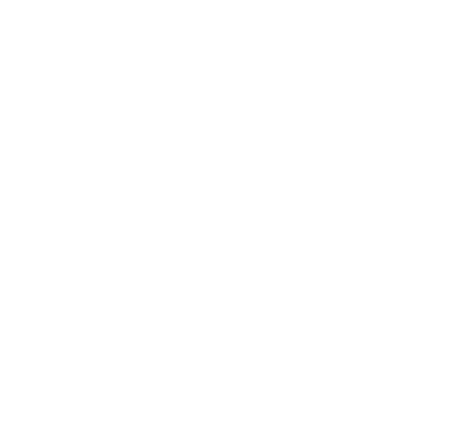2. Use the objective function to determine how many cookies of each type she should bake to maximize her daily profit. (2 marks)
9. The table below shows income tax rates in Kenya in a certain year.
 Monthly taxable income in Ksh Tax rate percentage % in each shilling Upto 16 680 10% From 16 681 to 28 900 15% From 28 901 to 51 920 20%
During the year, Lwande paid a net tax of Ksh. 5 449 after a relief of Ksh. 2 256 in a certain month. Calculate Lwande’s gross salary during the month if he enjoys a non-taxable travel allowance of Ksh. 15 000. (4 marks)
10. Towns A and B lie on the same latitude south of equator. When it is 2.20 p.m. at A, the time at B is 6.40 p.m. same day. Given that the longitude of town A is 30 Wº .
Find:
1. The longitude of town B. (1 mark)
2. The latitude where A and B lie to 1 decimal place given that the length of arc AB along the parallel of latitude is 2900 nautical miles. (2 marks)
11. A variable P varies jointly with the square of R and inversely with the square root of the difference between Q and S. If R is increased by 13% and both Q and S decreased by 20%. Find the percentage change in the value of P correct to 2 decimal places (3 marks)
12. The figure below is a distance – time graph of a certain object.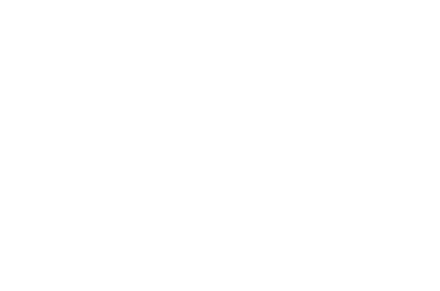From the graph, determine the average velocity of the object between t = 1 and t = 5 seconds. (2 marks)
13. The initial salary of Mr. Mbogo is Ksh. 52 000 per annum. His salary increases by 11% each year. Determine his total earnings after 13 years. Give your answer to the nearest thousands. (2 marks)
14. The figure below shows a cuboid of side 15 cm by 8 cm by 6 cm. M is the mid-point of AF.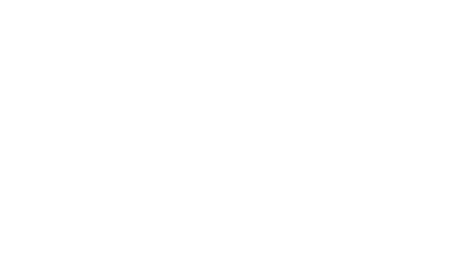Calculate the angle between HM and MB. (3 marks)
15. The current price of a car is Kshs.1 500 000. Given that its depreciation rate is r% p.a. and it took six years for its value to fall to Kshs 527 000. Calculate the value of r to the nearest whole number. (3 marks)
16. Vector r has a modulus of 3.5 and is parallel to vector s. Given that s = 6i - 2j - 3k, express r as a column vector. (2 marks)
SECTION II (50 marks)
Answer only five questions from this section in the spaces provided
17. The figure below is a circle centre O. ABT is a tangent to the circle at B and chord CD = 4.5 cm, BD = 4.0 cm and Dt = 8.0 cm.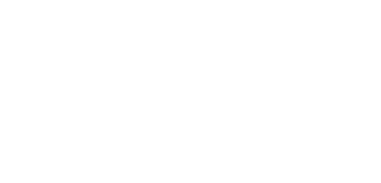Calculate:
1. The length of BT (2 marks)
2. The ∠DBT to the nearest ones (2 marks)
3. The radius of the circle correct to 2 decimal places (2 marks)
4. The area of the shaded region correct to 4 significant figures (4 marks)
18. A farmer has two tractors, A and B. The tractors working together can plough a farm in hours. One day the tractors started to plough the farm together. After one hour ten minutes, tractor B broke down but A continued alone and completed the job after a further six hours thirty minutes
Find:
1. The fraction of the job done by the tractors working together for one hour. (2 marks)
2. The fraction of the job done by tractor A after tractor B broke down. (3 marks)
3. The time each tractor working alone would have taken to plough the farm. (3 marks)
4. If the two tractors hired out would earn the farmer Ksh 90 000 after ploughing. Calculate how much money the farmer earned through tractor A. (2 marks)
19.
1. Complete the table below for the functions and to 1 d.p. for the range (2 marks)
 xº 0 15 30 45 60 75 90 105 120 135 150 165 y = 9/2cos(2x)º - 5/2 2 1.4 -2.5 -7.0 -2.5 1.4 y = 3sin(7/3x)º 0 1.7 1.9 -2.7 -2.9 1.3
2. Draw the graphs of y = 9/2(2x)º - 5/2 and y = 3sin(7/3x)º on the same grid. (4 marks)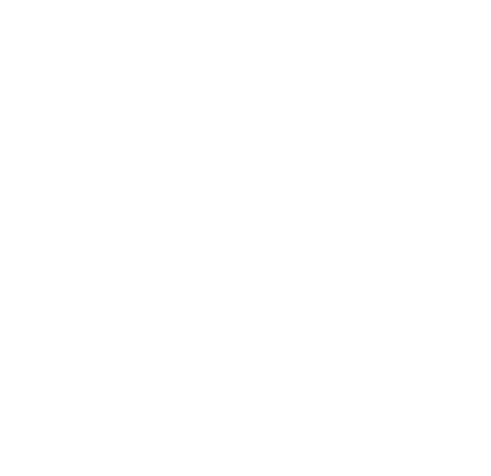3. Use your graph to solve
1. 9cos(2x) - 6sin(7/3x) = 5 (2 marks)
2. 9cos(2x) < -5 (2 marks)
20. Rectangle shown in which A(3,1), B(6,1), C(6,3) and D(3,3) undergoes a shear with y = 0 as the invariant line. A(3,1) is mapped onto A'(0,1)
1. On the grid provided, draw the image A'B'C'D' of rectangle ABCD under the shear. (3 marks)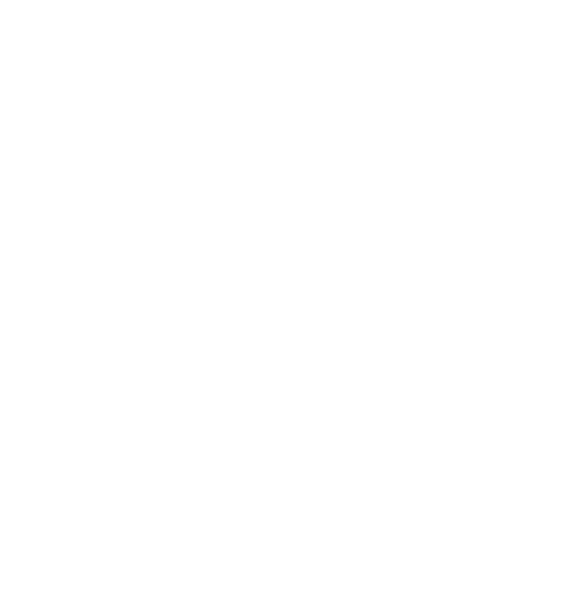2. Determine the matrix of the transformation. (2 marks)
3. The rectangle ABCD undergoes a transformation represented by the matrix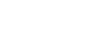to form a second image A''B''C''D''.
1. Find the coordinates of A''B''C''D'' (2 marks)
2. On the same grid draw the image A''B''C''D''(1 mark)
3. Describe the transformation T fully (2 marks)
21. A survey was conducted on 3000 students during a visiting day at Sunshine Senior School. Some of the probabilities calculated from the result are shown in the tree diagram below: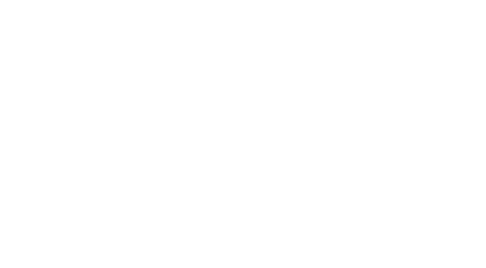1. How many boys in the survey were visited? (2 marks)
2. How many students in the survey were, visited but did not eat chicken and chips? (3 marks)
3. One of the students is selected at random. Find the probability that the student selected
1. Is not a visited girl and will eat chicken and chips (2 marks)
2. Is not visited and will eat chicken and chips (3 marks)
22. The heights of 100 maize plants were measured to the nearest centimeter and the results recorded and presented in the cumulative frequency curve below.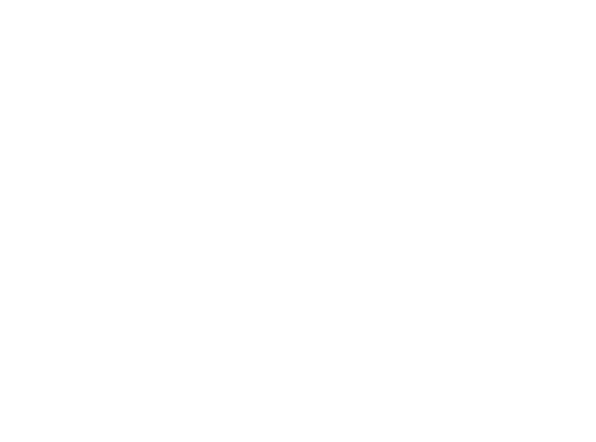1. Use the graph to:
1. Complete the table below for the column of class, c.f., and frequency (3 marks)
 Class c.f Frequency  y.f
2. Estimate the semi-interquartile range for the data. (3 marks)
2. Using a working mean of 43 ,calculate to 2 decimal places the standard deviation (4 marks)
23. The diagram below is a scale drawing of a piece of land. Three boundaries AB, AD and DC of the land are given. The fourth boundary is not given but it is known that the area of the land is greater than that of rectangle ABCD.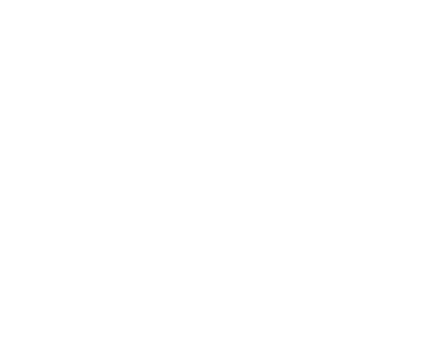Use a ruler and a pair of compasses only in this question
1. Construct the locus of all points equidistant from B and C. (1 mark)
2. The locus of any point P lying on the fourth boundary is such that ∠BPC = 120º. Draw the fourth boundary. (2 marks)
3. Shade the region within the land in which a variable point X must lie, given that X satisfies the following conditions:
1. X is at least 12 m from the boundary AB. (2 marks)
2. X is at least 22.5 m from A. (2 marks)
3. 60º ≤ DXC ≤ 90º (3 marks)
24. The figure below represents a sketch of the curve y = x2 - 3x - 10intersecting with the line y = 2x - 4 at points P and Q.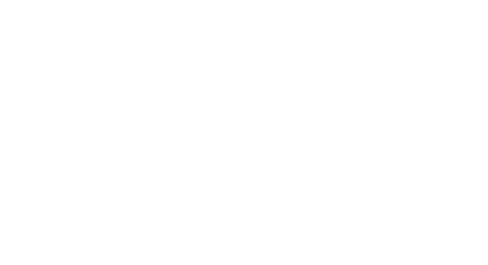Find:
1. The coordinates of points P and Q (3 marks)
2. The area of the shaded region. (7 marks)

• ✔ To read offline at any time.
• ✔ To Print at your convenience
• ✔ Share Easily with Friends / Students